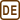## Thermal equilibrium of the Earth

Sun is the main source of energy for the Solar system. Let’s assume Sun radiates its energy as a black body with surface temperature of 5800 K. Radiation from the Sun spreads to all directions and supplies the energy to the Solar system. The average temperature of the planet is determined by the equilibrium of the absorbed solar energy and the energy radiated to the surroundings. What is the average temperature of Earth if we consider it to be a black body?

• #### Hint

Total intensity of the radiation I of black body, in our case of the Sun, is described by the Stefan-Boltzmann law:

$I=\sigma T^4,$

where σ = 5.67·10−8 Wm−2K−4. Intensity of the radiation is the power radiated from the unit area of the body.

Sun radiates energy in every direction equally therefore it is necessary to only determine how much of the radiated energy lands on the Earth’s surface.

Since we are considering equilibrium temperature of the Earth the same amount of energy which the Earth receives needs to be emitted from Earth too. Otherwise Earth will be either cooling down or heating up.

• #### Breakdown

According to the Stefan–Boltzmann law the intensity of radiation from a black body is proportional to the fourth power of its thermodynamic temperature, while the intensity is the total power of radiation divided by the area (surface of the Sun). From this we can determine the total amount of energy radiated from the Sun per unit of time.

In the same way we can determine the amount of energy which Earth will radiate while having its average temperature. In the equilibrium state this energy needs to be same as the one received by Earth from the Sun. Received energy can be determined from the distance between the Earth and the Sun and the size of the Earth.

• #### Notation

 T = 5800 K surface temperature of the Sun TE = ? (K) temperature of the Earth

From tables:

 R = 6.96·108 m Sun’s radius r = 1.50·1011 m distance between the Sun and the Earth RE = 6 380 km Earth’s radius
• #### Solution

Intensity of the radiation from a black body is described by the Stefan–Boltzmann law

$I=σT^4 ,$

where $$σ=5.67·10^{-8} \,\mathrm{Wm^{-2}}\,\mathrm{K^{-4}}$$ is the Stefan–Boltzmann constant. We will calculate the total radiation power P by multiplying by area S.

$P=SI .$

We will calculate the surface area S of the Sun from its radius R:

$S=4πR^{2} .$

Therefore the Sun radiates with power of

$P=4πR^{2}σT^{4} .$

This radiation spreads equally in all directions and its part PE lands on the Earth. Between the total power P and the power landing on Earth PE is the same ratio as between the area of the ball with radius r equal to the distance between Earth and the Sun r and the area that the Earth takes up from that area (meaning area of the circle with radius of the Earth RE). Therefore we have:

$\frac{P_{\mathrm{E}}}{P}=\frac{\pi R_{\mathrm{E}}^2}{4\pi r^2}\,\qquad \Rightarrow \qquad P_{\mathrm{E}}=4\pi r^2\sigma T^4\frac{\pi R_{\mathrm{E}}^2}{4\pi r^2}=\sigma T^4\frac{\pi R^2R_{\mathrm{E}}^2}{r^2}\,\mathrm{.}$

The power PE that Earth radiates can be determined in the same way as for the Sun but instead we use radius of the Earth RE and average thermodynamic temperature of the Earth TE

$P_{\mathrm{E}}=4πR_{\mathrm{E}}^{2}σT_{\mathrm{E}}^{4} .$

We will compare both formulas for PE:

$4\pi R_{\mathrm{E}}^2\sigma T_{\mathrm{E}}^4=\sigma T^4\frac{\pi R^2R_{\mathrm{E}}^2}{r^2}$

and determine the Earth’s temperature TE

$T_{\mathrm{E}}^4=T^4\frac{R^2}{4r^2}$ $T_{\mathrm{E}}=T\sqrt{\frac{R}{2r}}\,\mathrm{.}$

Using the Sun’s temperature T = 5800 K, Sun’s radius R = 6.96·108 m and the distance between the Earth and the Sun r = 1.50·1011 m, we get

$T_{\mathrm{E}}=5800\,\sqrt{\frac{6.96{\cdot}10^8}{2{\cdot}1.50{\cdot}10^11}}\,\mathrm{K}=279\,\mathrm{K}=6\,\mathrm{^{\circ}C\,.}$